•  例如: CV_8UC1 表示8位无符号单通道矩阵, CV_32SC2表示32位符号双通道矩阵.  例: CvMat* M = cvCreateMat(4,4,CV_32FC1); 9）逐点赋值方式初始化 CvMat* mat = cvCreateMat( 2, 2,CV_64...
1)结构体IplImage
OpenCv中图像的结构体为IplImage，位于头文件cxcore.h中，IplImage结构体的定义如下：

typedef struct _IplImage
{
int nSize;
/* IplImage大小 */
int ID;
/* 版本 (=0)*/
int nChannels;
/* 大多数OPENCV函数支持1,2,3 或 4 个通道 */
int alphaChannel;
/* 被OpenCV忽略 */
int depth;
/* 像素的位深度，主要有以下支持格式：
IPL_DEPTH_8U, IPL_DEPTH_8S,IPL_DEPTH_16U,IPL_DEPTH_16S, IPL_DEPTH_32S,

IPL_DEPTH_32F 和IPL_DEPTH_64F */
char colorModel;
/* 被OpenCV忽略 */
char channelSeq; /* 同上 */
int dataOrder;
/* 0 - 交叉存取颜色通道, 1 - 分开的颜色通道.

只有cvCreateImage可以创建交叉存取图像 */
int origin;
/*图像原点位置： 0表示顶-左结构,1表示底-左结构 */
int align;
/* 图像行排列方式 (4or 8)，在 OpenCV 被忽略，
使用 widthStep 代替 */
int width;
/* 图像宽像素数 */
int height;/* 图像高像素数*/
struct _IplROI *roi;/* 图像感兴趣区域，当该值非空时，

只对该区域进行处理 */
struct _IplImage *maskROI; /* 在 OpenCV中必须为NULL */
void *imageId;
/* 同上*/
struct _IplTileInfo *tileInfo; /*同上*/
int imageSize;
/* 图像数据大小(在交叉存取格式下ImageSize=
image->height*image->widthStep），单位字节*/
char *imageData;
/* 指向排列的图像数据 */
int widthStep;
/* 排列的图像行大小，以字节为单位 */
int BorderMode;/* 边际结束模式, 在 OpenCV 被忽略*/
int BorderConst;
/* 同上 */
char *imageDataOrigin; /* 指针指向一个不同的图像数据结构
（不是必须排列的），是为了纠正图像内存分配准备的 */
} IplImage;

主要的成员变量有。
nChannels : 图像的通道数目，即灰度图像：nChannels= 1; RGB图像nChannels= 3
depth:每个像素值的数据类型和所占的存储空间
origin变量可以有两种取值：IPL_ORIGIN_TL 或者 IPL_ORIGIN_BL，分别设置坐标原点的位置于图像的左上角或者左下角。在计算机视觉领域，一个重要的错误来源就是原点位置的定义不统一。具体而言，图像的来源、操作系统、编解码器和存储格式等因素都可以影响图像坐标原点的选取。举例来说，你或许认为自己正在从图像上面的脸部附近取样，但实际上却在图像下方的裙子附近取样。避免此类现象发生的最好办法是在最开始的时候检查一下系统，在所操作的图像块的地方画点东西试试。
dataOrder:   多通道的数据存储方式，dataOrder=0是交叉通道存储方式，即BGRBGRBGRBGR的方式存储；dataOrder=1是采用独立通道方式存储，即RRRRRRR。。。，GGGGGGG…,BBBBBB…,一般都是BGRBGRBGR的这种交叉存储方式，cvCreateImage生成的图像也是这种存储方式。
width:      图像的宽度
height:      图像的高度
imageData:  图像的像素矩阵
widthStep:   每一行像素所占的字节数目. 参数widthStep包括相邻行的同列点之间的字节数。仅凭变量width是不能计算这个值的，因为为了处理过程更高效每行都会用固定的字节数来对齐；因此在第i行末和第i+1行开始处可能会有些冗于字节。参数imageData包含一个指向第一行图像数据的指针。如果图像中有些独立的平面(如当dataOrder
= IPL_DATA_ORDER_PLANE)那么把它们作为单独的图像连续摆放，总行数为height和nChannels的乘积。但通常情况下，它们是交错的，使得行数等于高度，而且每一行都有序地包含交错的通道。
ROI-- 感兴趣的区域(ROI)，实际上它是另一个IPL/IPP 结构IplROI的实例。IplROI包含xOffset，yOffset，height，width和coi成员变量，其中COI代表channel of interest(感兴趣的通道)。ROI的思想是： 一旦设定ROI，通常作用于整幅图像的函数便会只对ROI所表示的子图像进行操作。如果IplImage变量中设置了ROI，则所有的OpenCV函数就会使用该ROI变量。如果COI被设置成非0值，则对该图像的操作就只作用于被指定的通道上了
。不幸的是，许多OpenCV函数都忽略参数COI。
IplImage*cvLoadImage( const char* filename, int iscolor);
其中，filename是待载入图像的名称，包括图像的扩展名；iscolor是一个辅助参数项，可选正数、零和负数三种值，正数表示作为三通道图像载入，零表示该图像作为单通道图像，负数表示载入图像的通道数由图像文件自身决定。

3）窗口定义函数  cvNamedWindow
函数cvNamedWindow定义一个窗口，用于显示图像。其函数原型如下：
int cvNamedWindow( const char* name, unsignedlong flags );
其中，name是窗口名，flags是窗口属性指标值，可以选择CV_WINDOW_AUTOSIZE和0两种值。CV_WINDOW_AUTOSIZE表示窗口尺寸与图像原始尺寸相同，0表示以固定的窗口尺寸显示图像。

4）图像显示函数 cvShowImage

函数cvShowImage是在指定的窗口中显示图像，其函数原型如下：
void cvShowImage( const char* name, constCvArr* image );
其中，name是窗口名称，image是图像类型指针，一般是IplImage指针。
5）图像保存函数 cvSaveImage

函数cvSaveImage以指定的文件名保存IplImage类型的指针变量，其函数原型如下：
int cvSaveImage( const char* filename, constCvArr* image );
其中，filename是图像保存路径和名称，image是IplImage指针变量。
Trick：
如果要保存一组图像到result文件夹,图像个数为n,保存名称按照一定的序号递增，假设为imgTmp0.jpg，imgTmp1.jpg，imgTmp2.jpg，imgTmp3.jpg，…, imgTmpn.jpg，则
操作为：
char* f;
for(inti=0; i<n; i++)
{
sprintf(f,”result/imgTmp%d.jpg”,i);//把格式化的数据写入某个字符串缓冲区f中
cvSaveImage(f,img);
}
借用sprintf函数即可以完成依次命名的功能。
6）图像销毁函数  cvReleaseImage

函数cvReleaseImage销毁已定义的IplImage指针变量，释放占用内存空间。其函数原型如下：
void cvReleaseImage( IplImage** image );
其中，image为已定义的IplImage指针。
7）矩阵结构体 CvMat

CvMat的结构体定义为：
typedef struct CvMat
{
int type; /* CvMat signature (CV_MAT_MAGIC_VAL), element type and flags */
int step; /* full row length in bytes */
int* refcount; /* underlying data reference counter */
union
{
uchar* ptr;//数据头指针
short* s;
int* i;   //int,pMat->data.i;
float* fl;//float,pMat->data.fl;
double* db;
} data; /* data pointers */

#ifdef __cplusplus
union
{
int rows;
int height;
};
union
{
int cols;
int width;
};
#else
int rows; /* number of rows */
int cols; /* number of columns */
#endif

} CvMat;
step是每一行数据的长度，以字节来表示
data是存放矩阵数据的联合体，如果矩阵pMat是float类型的，那么获取矩阵数据指针的方式为pMat->data.fl，如果是整型的 pMat->data.i
行数和列数在c和c++中定义略有不同，但是rows和cols是通用的两个变量。
cvMat：
CV_INLINE  CvMat  cvMat( int rows, int cols, int type, void* data CV_DEFAULT(NULL))
{
CvMat m;
assert( (unsigned)CV_MAT_DEPTH(type) <= CV_64F );
type = CV_MAT_TYPE(type);
m.type = CV_MAT_MAGIC_VAL | CV_MAT_CONT_FLAG | type;
m.cols = cols;
m.rows = rows;
m.step = m.cols*CV_ELEM_SIZE(type);
m.data.ptr = (uchar*)data;
m.refcount = NULL;
m.hdr_refcount = 0;
return m;
}
8）分配矩阵空间   cvCreateMat

CvMat* cvCreateMat(int rows, int cols, int type);

type: 矩阵元素类型. 格式为CV_<bit_depth>(S|U|F)C<number_of_channels>.
例如: CV_8UC1 表示8位无符号单通道矩阵, CV_32SC2表示32位有符号双通道矩阵.

例:  CvMat* M = cvCreateMat(4,4,CV_32FC1);

9）逐点赋值方式初始化

CvMat* mat = cvCreateMat( 2, 2,CV_64FC1 );
cvZero( mat );
cvmSet( mat, 0, 0, 1 );
cvmSet( mat, 0, 1, 2 );
cvmSet( mat, 1, 0, 3 );
cvmSet( mat, 2, 2, 4 );
cvReleaseMat( &mat );
10）使用现有数组初始化

doublea[] = { 1, 2, 3, 4,
5, 6, 7, 8,
9, 10, 11, 12 };
CvMat mat = cvMat( 3, 4, CV_64FC1, a ); // 64FC1 for double// 不需要cvReleaseMat，因为数据内存分配是由double定义的数组进行的。
或者这样：
float vals[]={0.866000,0.555000,0.333000,0.222000};
CvMat rotmat;

11）释放矩阵

CvMat* M = cvCreateMat(4,4,CV_32FC1);
cvReleaseMat(&M);//矩阵指针的地址
或者 void cvReleaseMat(CvMat  * * mat);//矩阵指针的指针

12）复制矩阵

CvMat* M1 = cvCreateMat(4,4,CV_32FC1);
CvMat* M2;
M2=cvCloneMat(M1);

13）存取矩阵元素
a,从矩阵中得到一个元素的最简单的方法是利用宏CV_MAT_ELEM()。

例1,利用CV_MAT_ELEM()宏存取矩阵
1.  CvMat* mat = cvCreateMat( 5, 5, CV_32FC1 );
2.  float element_3_2 = CV_MAT_ELEM( *mat, float, 3, 2 );

b,还有一个与此宏类似的宏，叫CV_MAT_ELEM_PTR()。CV_MAT_ELEM_
PTR()传入矩阵、待返回元素的行和列号这3个参数，返回指向这个元素的指针。

例2：利用宏CV_MAT_ELEM_PTR()为矩阵设置一个数值
1.  CvMat* mat = cvCreateMat( 5, 5, CV_32FC1 );
2.  float element_3_2 = 7.7;
3.  *( (float*)CV_MAT_ELEM_PTR( *mat, 3, 2 ) ) = element_3_2;

c,例3：指针访问矩阵结构
1.  uchar* cvPtr1D(
2.    const CvArr*  arr,
3.    int           idx0,
4.    int*          type = NULL
5.  );
6.
7.  uchar* cvPtr2D(
8.    const CvArr*  arr,
9.    int           idx0,
13.   int           idx1,
14.   int*          type = NULL
15. );
16.
17. uchar* cvPtr3D(
18.   const CvArr*  arr,
19.   int           idx0,
20.   int           idx1,
21.   int           idx2,
22.   int*          type = NULL
23. );
24. uchar* cvPtrND(
25.   const CvArr*  arr,
26.   int*          idx,
27.   int*          type            = NULL,
28.   int           create_node     = 1,
29.   unsigned*     precalc_hashval = NULL
30. );

d,如果仅仅是读取数据，可用另一个函数族cvGet*D。但是返回矩阵元素的实际值。

例4：CvMat和IPlImage元素函数
1.  double cvGetReal1D( const CvArr* arr, int idx0 );
2.  double cvGetReal2D( const CvArr* arr, int idx0, int idx1 );
3.  double cvGetReal3D( const CvArr* arr, int idx0, int idx1, int idx2 );
4.  double cvGetRealND( const CvArr* arr, int* idx );
5.
6.  CvScalar cvGet1D( const CvArr* arr, int idx0 );
7.  CvScalar cvGet2D( const CvArr* arr, int idx0, int idx1 );
8.  CvScalar cvGet3D( const CvArr* arr, int idx0, int idx1, int idx2 );
9.  CvScalar cvGetND( const CvArr* arr, int* idx );

e,例5：为CvMat或者IplImage元素设定值的函数

1.  void cvSetReal1D( CvArr* arr, int idx0, double value );
2.  void cvSetReal2D( CvArr* arr, int idx0, int idx1, double value );
3.  void cvSetReal3D(
4.    CvArr* arr,
5.    int idx0,
6.    int idx1,
7.    int idx2,
8.    double value
9.  );
10. void cvSetRealND( CvArr* arr, int* idx, double value );
11.
12. void cvSet1D( CvArr* arr, int idx0, CvScalar value );
13. void cvSet2D( CvArr* arr, int idx0, int idx1, CvScalar value );
14. void cvSet3D(
15.   CvArr* arr,
16.   int idx0,
18.   int idx1,
19.   int idx2,
20.   CvScalar value
21. );
22. void cvSetND( CvArr* arr, int* idx, CvScalar value );

为了方便，我们也可以使用cvmSet()和cvmGet()，这两个函数用于处理浮点型单通道矩阵，非常简单。
1.  double cvmGet( const CvMat* mat, int row, int col )
2.  void cvmSet( CvMat* mat, int row, int col, double value )
以下函数调用cvmSet()：
1.  cvmSet( mat, 2, 2, 0.5000 );
等同于cvSetReal2D函数调用：
1.  cvSetReal2D( mat, 2, 2, 0.5000 );

f,例6：累加一个三通道矩阵中的所有元素
1.  float sum( const CvMat* mat )
{
3.
float s = 0.0f;
4.
for(int row=0; row<mat->rows; row++ )
{
const float* ptr=(const float*)(mat->data.ptr + row * mat->step);
6.
for( col=0; col<mat->cols; col++ )
{
7.
s += *ptr++;
8.
}
10.   }
11.   return( s );
12. }

g,间接存取矩阵元素

cvmSet(M,i,j,2.0); // Set M(i,j)
t = cvmGet(M,i,j); // Get M(i,j)

注意：cvmGet()和cvmSet（）函数只支持CV_32FC1(float)和CV_64FC1(double)的类型

h,直接存取，使用4字节校正

CvMat* M = cvCreateMat(4,4,CV_32FC1);
int n = M->cols;
float *data = M->data.fl;//注意:是浮点型数据

data[i*n+j] = 3.0;

i,直接存取，使用任意字节

CvMat* M = cvCreateMat(4,4,CV_32FC1);
int step = M->step/sizeof(float);
float *data = M->data.fl;

(data+i*step)[j] = 3.0;

j,直接存取一个初始化的矩阵

double a;
CvMat Ma = cvMat(3, 4, CV_64FC1, a);
a[i*4+j] = 2.0; // Ma(i,j)=2.0;

14，矩阵/向量数学操作
a,矩阵-矩阵操作

CvMat *Ma, *Mb, *Mc;
cvAdd(Ma, Mb, Mc);            // Ma+Mb -> Mc
cvSub(Ma, Mb, Mc);            // Ma-Mb -> Mc
cvMatMul(Ma, Mb, Mc);         // Ma*Mb -> Mc

b,矩阵元素操作

CvMat *Ma, *Mb, *Mc;
cvMul(Ma, Mb, Mc);            // Ma.*Mb -> Mc
cvDiv(Ma, Mb, Mc);            // Ma./Mb -> Mc
cvAddS(Ma, cvScalar(-10.0), Mc); // Ma.-10 -> Mc

c,向量乘积

double va[] = {1, 2, 3};
double vb[] = {0, 0, 1};
double vc;

CvMat Va=cvMat(3, 1, CV_64FC1, va);
CvMat Vb=cvMat(3, 1, CV_64FC1, vb);
CvMat Vc=cvMat(3, 1, CV_64FC1, vc);

double res=cvDotProduct(&Va,&Vb); // 点乘:  Va . Vb -> res
cvCrossProduct(&Va, &Vb, &Vc);    // 向量积: Va x Vb -> Vc

注意 Va, Vb, Vc 在向量积中向量元素个数须相同.

d,单矩阵操作

CvMat *Ma, *Mb;
cvTranspose(Ma, Mb);   // transpose(Ma) -> Mb (不能对自身进行转置)
CvScalar t = cvTrace(Ma); // trace(Ma) -> t.val
double d = cvDet(Ma);     // det(Ma) -> d
cvInvert(Ma, Mb);         // inv(Ma) -> Mb

e,非齐次线性系统求解

CvMat* A  = cvCreateMat(3,3,CV_32FC1);
CvMat* x  = cvCreateMat(3,1,CV_32FC1);
CvMat* b  = cvCreateMat(3,1,CV_32FC1);
cvSolve(&A, &b, &x);          // solve (Ax=b) for x

f,特征值分析（对称矩阵）

CvMat* A  = cvCreateMat(3,3,CV_32FC1);
CvMat* E  = cvCreateMat(3,3,CV_32FC1);
CvMat* l  = cvCreateMat(3,1,CV_32FC1);
cvEigenVV(&A, &E, &l);        // l = A的特征值 (降序排列)
// E = 对应的特征向量 (每行)

g,奇异值分解

CvMat* A  = cvCreateMat(3,3,CV_32FC1);
CvMat* U  = cvCreateMat(3,3,CV_32FC1);
CvMat* D  = cvCreateMat(3,3,CV_32FC1);
CvMat* V  = cvCreateMat(3,3,CV_32FC1);
cvSVD(A, D, U, V, CV_SVD_U_T|CV_SVD_V_T); // A = U D V^T

标号使得 U 和 V 返回时被转置(若没有转置标号，则有问题不成功!!!).


展开全文opencv
• 矩阵对角化引入探讨线性变换的对角化问题。（定义、等价命题和定理）
矩阵的相似标准型之“可对角化的条件”

本节主要围绕着矩阵（或线性变换）能否进行对角化以及如何进行对角化进行讨论。
【对角化的判断】

矩阵的对角化：对给定的矩阵，判断能否相似于对角阵
线性变换的对角化：对给定的线性空间上的线性变换，判断是否存在空间的一组基，使得其矩阵是对角阵。

前面有关线性变换、线性空间和矩阵讨论了那么多，我们已经可以在矩阵和线性变换之间建立一个对应关系了，因此矩阵的对角化问题和相似变换的对角化问题在某种程度上是一致的。

一. 矩阵对角化(回顾)

矩阵对角化↔矩阵相似于对角阵

以下回顾线性代数中三个相似于对角阵的判定定理

定理1——线性无关的特征向量：上述过程理解的时候可以按照从左边推向右边来理解，但是其变化都是等价变化，也就是两边都可以互相推导。
：矩阵A相似于对角阵的定义——存在一个可逆阵P，有P-1·A·P = Λ成立，而且根据“相似的矩阵具有相同的特征量”，可以推知对角矩阵Λ的对角线上的元素应该就是各个特征值。
：将矩阵P拆成列分块矩阵，对等式P-1·A·P = Λ两边同时左乘矩阵P，就能得到A·P = P·Λ

小结：前面几节中一些推导证明中老师也用过这样的变化技巧，以后看到形如P-1AP这样的式子，就可以考虑左乘P-1的逆矩阵来变换。

：分块矩阵的运算
：根据等式，可以得到APi = λiPi（i = 1,2,…,n）的一系列等式，根据特征值和特征向量的定义，Pi就是矩阵A对应于特征值λi的特征向量。又因为Pi是P矩阵的列分块且矩阵P可逆，“可逆矩阵的列(行)向量组都是线性无关的”，因此得到——A有n个线性无关的特征向量。

通过定理一，我们知道要判断某个矩阵能否进行对角化，只需要计算矩阵有多少个线性无关的特征向量。

问题是我们如何快速判断矩阵的特征向量是否线性无关呢？——引出定理2.

定理2——特征向量的线性无关性由此，将判断特征向量是否线性无关的问题转化成求解特征值，并判断是否具有n个互异的特征值。

定理3——重根的特征向量的线性无关性该定理告诉我们，每个特征值的线性无关的特征向量组合在一起构成的特征向量组依然是线性无关的。

二. 线性变换的可对角化问题

把关于矩阵对角化的定理嫁接到线性变换的问题讨论中来。
读者可以将第一和第二部分的定理描述与证明对照起来理解、记忆。

已知——假设V是n维线性空间，f∈End(V):
定理1<必要性证明>：对于这条定理的证明我们有两条思路。其一，就是把线性变换用矩阵语言描述出来，然后通过矩阵的对角化条件进行证明；其二，我们可以直接用线性变换的语言来进行描述和证明。这里我们采用第二种方法。
：线性变换f可以对角化的定义——存在一组基，使得变换f在这组基下的矩阵为对角阵。
p.s. 这里对角阵中对角线上的元素现在并不能确定为特征值，只是用λ这个符号表示而已。
：线性变换的矩阵表示，已知一个向量ε和线性变换对应的矩阵A，那么该原像对应的像应该是Aε。
：根据线性变换的特征值和特征向量的定义，且εi≠0(i = 1,2,…,n)，所以λi就是线性变换f 的特征值，εi是线性变换f对应于特征值λi的特征向量。

线性变换的特征向量的定义和一些基本介绍参考博文《【矩阵论】矩阵的相似标准型（1）》：因为ε1，ε2，…，εn本来就是取的一组基，所以该向量组肯定是线性无关的。从而得证f有n个线性无关的特征向量。
<充分性证明>：已知条件，f有n个线性无关的特征向量η1，η2，…，ηn，且满足特征向量的定义
：因为f是n维线性空间V中的一个线性变换，且向量组η1，η2，…，ηn是n个线性无关的特征向量。“n维空间中任意n个线性无关的特征向量都是该n维空间的一组基”，因此V的基就是η1，η2，…，ηn。
：求解线性变换f在给定的一组基η1，η2，…，ηn下的矩阵表示，就是要求解原像ηi(i = 1,2,…,n)经过变换后得到的像f(ηi)在该组基下的坐标。且我们又已知f(ηi) = λiηi，所以能够写出来矩阵就是一个对角阵。

同样地，与矩阵对角化讨论思路一样，我们需要解决特征向量是否线性无关的问题，引出了定理二。

定理2证明“线性相关性”题目的时候往往是根据定义，对于一组向量αi,写出一组线性方程Σki ·αi = 0，然后判断这个方程是否具有非零解。按照线性相关性的定义写出x1η1+x2η2+…+xsηs = θ，按照线性变换f的线性性可以展开得到上式(1)，等式两边同时乘上λ1即可得到上式(2)
（1）-（2）得到下列方程思路：【归纳假设】
因为我们最终是要证明这s个特征向量线性无关，可以采用数学归纳法。

①当k = 1时，ηk = η1≠θ，一定是线性无关的；
②假设当k = s-1时，η1，η2，…，ηs-1是线性无关的；
③则需要证明当k = s时，η1，η2，…，ηs-1，ηs也是线性无关的。

则在假设的前提下，上图中s-1个特征向量，η2，…，ηs应该是线性无关的，所以各项系数应该全为0.
又因为特征值是互异的，所以只可能有x2 = x3 = … = xs = 0
将x2 = x3 = … = xs = 0代入到x1η1+x2η2+…+xsηs = θ，则有x1η1 = θ，所以能推出x1 = 0，最终推出这s个向量都是线性无关的。

至此，当我们判断一个线性变换是否可对角化的时候，只需要判断这个线性变换是否具有n个线性无关的特征向量；

要判断这n个特征向量是否线性无关，只需要确定其对应的n个特征值是不是互异的。

定理3同样地，各组线性无关的特征向量组合在一个依然是一组线性无关的特征向量。
略去不证。

三. 特征子空间

对于线性无关特征向量的个数这一概念，还可以借助“特征子空间”进行描述。

1. 定义
（1）f 的相应于特征值 λ0 的特征子空间【为什么V0一定是子空间】

对于f(η) = λ0·η，可以把这一线性空间看成是【f-λ0·I】的核子空间，即Ker(f-λ0·I)，其中I是恒等变换。

把核子空间的定义代入，Ker(f-λ0·I) = {η|f-λ0·I = θ} = {η|f = λ0·I }，也就是说f的作用就是使原像在数值上扩大或缩小λ0倍，满足这样关系的原像必然满足f(η) = λ0η的关系式。

【V0中不止包含f线性变换对应于λ0的特征向量】

V0是一个子空间，那么零向量θ肯定是要在子空间中的。
我们可以将θ代入进行验证，f(θ) = λ0θ = θ，所以θ∈V0。

综上，Vλ0里面所含的向量应该是线性变换f关于λ0的特征向量以及零向量。

（2）重要性
我们之前提起过，引出“特征子空间”这一概念是为了对【线性无关的特征向量的个数】进行描述。
基于此，f 的属于 λ0 的线性无关的特征向量的个数 = dim（Vλ0）
2. 例题演练
【例】 求解给定的线性变换的特征值和特征子空间求解线性变换的特征值，就是把线性变换关于某一组基的矩阵求解出来，然后求解该矩阵的特征值。

本题所示的这个线性变换的特征多项式和特征值我们在《【矩阵论】矩阵的相似标准型（1）》中求解过了

部分演算如下图所示，其特征值是一个四重根——0则我们要求的V0 = { η | f(η) = θ } = Ker(f)
在该系列第(1)篇博文中我们对线性变换关于特征值0的特征向量也都进行了求解，形如 a[[1,0],[-1,0]]+b[[0,1],[0,-1]]，其中a和b不全为0。
也就是说V0这个空间是由[[1,0],[-1,0]]和[[0,1],[0,-1]]这两个向量向量张成的子空间，因此要求解V0的维数，只需要验证该两个向量构成的向量组的秩。
显然，这两个向量线性无关，所以特征子空间的维数为2，基就是该向量组。
3. 定理1——特征子空间的维数不超过特征根的重数
（1）定理描述关于特征值的代数重数和几何重数

ri：【代数重数】，对于特征方程进行代数求解得到的特征根的重数。

dim Vλi：【几何重数】，关于特征值λi的特征子空间的维数，从几何的角度去观察。

（2）定理证明

【方法论】
碰到有子空间、线性变换与矩阵这样类型的证明过程时，老师有几个惯用的证明技巧：
①将子空间的一组基进行拓展，得到更大的一个空间的一组基

②针对扩展出来的基，把线性变换的矩阵表示求解出来
p.s. 此时这个求解出来的矩阵往往具有较好的运算性质，比如是对角或者是上三角矩阵之类的。

对于线性变换f，任取一个特征值λ0，设f对应于该特征值的特征子空间的维数（几何重数）为t，则构造出该特征子空间的一组基。
通过线性无关向量组的拓展，可以把特征子空间Vλ0的一组基ε1，ε2，…，εt扩展成线性变换所在的线性空间V的一组基ε1，ε2，…，εt，εt+1，εt+2，…，εn接下来关于这一组基ε1，ε2，…，εt，εt+1，εt+2，…，εn，求解线性变换 f 的矩阵表示：

【强调一下】
在某一组基下求解线性变换f的矩阵表示时，打好框架——

(f(ε1)，f(ε2)，…，f(εn)） = （ε1，ε2，…，εn）·A

打好框架后，对于矩阵A，可以每一列每一列地单独考虑。
其第一列就应该是f(ε1)这个变换后的像在基ε1，ε2，…，εn下的坐标表示。
因为ε1在f关于λ0的特征子空间之中，所以具有f(ε1) = λ0ε1的关系，故第一列只有第一个元素为λ0，其余均为0，剩下t列（均在特征子空间中）以此类推。
剩下的n-t列，我们不需要额外关注，故写成下图形式。既然得到了矩阵A的形式，那么我们要利用这个矩阵来求解线性变换f的特征多项式。
按照分块矩阵行列式的运算规则，可知道|λI-A|中至少包含t个(λ-λ0)的因子。(分块矩阵的左上角分块)
因此就证明出特征值λ0的几何重数t是不大于特征值的代数重数的。4. 定理2——线性变换f可对角化的判定条件
（1）定理描述（2）定理证明

对于一系列等价条件的证明，往往采用循环证明的方法。

【1→2】
假设线性空间V是n维的，那么对于线性变换f∈End（V）,f的特征多项式一定是n次的。
故：r1+r2+…+rs = n
又：f是可以对角化的。
根据线性变换可对角化的三个性质中的第三点，可以知道f一定有n个线性无关的特征向量。
结合代数重数与几何重数之间的不等关系与等式的夹逼，就能由定理1推得定理2.【2→3】

【方法论】
证明某个集合是另一组集合的直和，需要考虑两个问题：
其一，另一组集合的和运算是否为直和
其二，左边的集合和另一组集合和运算的结果是否相等。

Vλ1+Vλ2+…+Vλs是直和

按照往常我们要证明直和，通常都会采用这些集合的交空间为零空间来证明。

但是对于特征子空间，我们有更方便利用的性质定理。

根据前面的定理，我们知道，在各个Vλi中找到一组线性无关的特征向量(i= 1,2,…,s)，然后把它们合并在一起，依然是一组线性无关的向量。
换言之，如果在各个特征子空间中找到它们的基，它们的基合并起来也应该就是Vλ1+Vλ2+…+Vλs对应的基，这一点就满足了直和运算的判定命题。
V = Vλ1+Vλ2+…+Vλs

按照往常证明两个集合相等往往是采用两个集合的相互包含性。

这里因为若干特征子空间的和一定也是V空间的子空间，证明一个空间和其子空间相等只需要证明两个空间的维度相等即可。：已经证明了Vλ1+Vλ2+…+Vλs是直和，所以直和的空间维度等于各个部分空间的维度之和
：根据第2点的已知条件dimVλi = ri
：根据题干中特征多项式的表示，重根的重数之和就应该等于原空间V的维数。
证毕。
【3→1】
根据空间的直和运算，可以把每一个特征子空间的基列出来，这些基拓展在一块儿就构成了线性空间V的基。
把线性变换f在V的这组基下的矩阵表示写出来，如下图所示，显然是一个对角阵。
故证明出线性变换f的可对角化。（3）意义
三条等价定理的证明已经结束，如果我们再返回去看看这三条定理的本质，其实和矩阵可对角化的三条等价定理是一致的。
第二条——说明了每个特征值对应的特征向量有多少个是线性无关的；
第三条——说明了每个特征值的无关特征向量可以组合在一起。
p.s. 只不过用“直和”这种描述方式更显得清楚。

【例】线性变换的系列题目

系列题目：
线性变换的矩阵表示/
线性变换的特征量与特征子空间的求解/
线性变换可对角化的判断<解>

打好矩阵求解框架——求解各个像在选定的基下的坐标表示特征值→特征多项式；
特征子空间的基→相应于某一特征值线性无关的特征向量下面求特征子空间的基时要注意：
将特征值代入之后，需要求解一个矩阵方程。

矩阵方程求出来的基础解系并不一定就是需要的特征子空间的基，基础解系只是在我们所选定的基下的坐标表示，还要把坐标和基一起运算才能得到最终的向量——所求的向量才是特征子空间的基。3. 线性变换的对角化判定
①代数重数 = 几何重数
dim V0 = 2;dim V3 = 2;
②线性无关的特征向量的个数
在V0和V3特征子空间中均分别找出了2个线性无关的特征向量，一共具有4个线性无关的特征向量且空间C2x2在选定的基表示下也是四维的。
③基的拓展性
将V0和V3特征子空间的基拿出来拓展在一起，就是C2x2空间的基。

5. 定理3——线性变换可对角化的充要条件
（1）定理描述（2）定理证明
①引理及证明.该引理证明的核心就在于反复利用矩阵乘积的秩的不等式：

r(Asxn·Bnxt) ≥ r(A) + r(B) - n②定理证明
【必要性】
按照矩阵相似于对角阵的定义，可以得到其中，对角阵是若干个分块对角矩阵，有ri个λi,i = 1,2,…s。
前几篇文章我们讨论了很多有关化零多项式、最小多项式的相关内容。我们知道，相似的两个矩阵具有相同的最小多项式。
因此，想要证明矩阵A的最小多项式没有重根，只需要证明与矩阵A相似的对角矩阵Λ的最小多项式没有重根即可。
矩阵Λ的可能的最小多项式形式为：且经过验证，该多项式次数最低且是化零多项式，它就是我们需要的最小多项式。因为λi（i=1,2,…,s）是互不相同的特征值，所以显然该最小多项式是没有重根的。
【充分性】
现已知A的最小多项式没有重根，则可以把A的最小多项式写出如下：要能够证明矩阵A相似于对角阵，矩阵对角化的判定定理和性质很有多，我们选择证明——矩阵A有n个线性无关的特征向量。

根据已知直到A有s个互异的特征值，只要求出对应每一个特征值有多少线性无关的特征向量，这些无关向量的个数总和为n即可。

按照特征值和特征向量的计算方法，对于特征值λi，需要求解齐次线性方程组（A-λi·I）x = θ，求解出其的基础解系中有多少个向量，则对应就有多少个线性无关的特征向量。
熟悉矩阵的列空间和左零空间的关系的话，对于一个n阶方阵，其列空间的秩如果为r，则左零空间的秩就为n-r；
且左零空间的秩就是齐次线性方程组基础解系中向量的个数。现在需要求解Σr(A-λiI)的数值，用到我们前面证明过的引理。

因为最小多项式也是化零多项式，所以代入矩阵A进入mλ表达式，即有：

(A-λ1I)·(A-λ2I)…(A-λsI) = O，所以Σr(A-λiI)≤(s-1)n
回代入sn-Σr(A-λiI)≥n；又因为矩阵A是n阶的，其含有的无关特征向量不会超过n。

综上，得证A具有n个线性无关的特征向量→A是相似于对角阵的。

【例】-1 证明矩阵可对角化

矩阵的最小多项式没有重根→矩阵可对角化，这是很方便也很好用的一个证明性质。以上，可以根据矩阵的等式关系得到矩阵的化零多项式，且该化零多项式无重根。
又已知矩阵的最小多项式可以整除化零多项式，那么矩阵的最小多项式就更加没有重根了。
从而能够证明出矩阵可以对角化。
【例】-2 已知一个矩阵方程，求解矩阵相关的行列式

已知一个有关矩阵的方程，可以挖掘出诸如化零多项式、特征量等很多信息。

Tips:另外在有“相似”关系的问题中，常用到形如P-1AP这样的运算式，这就是利用了矩阵的相似关系。
相似的矩阵往往会具有很多的相同量——如特征值、特征多项式等等。
且P是一个可逆矩阵，这对于行列式运算也有很大的简化作用。

根据前面的定理，确定了矩阵A可以对角化，且也确定了其相似的对角阵的一般形式根据已知条件 r(A-5I) = r，因为相似的矩阵具有相同的秩，所以对于(A-5I)进行相似变换，得到Λ-5I这样的矩阵；
Λ的形式之前已经确定了如上图，再减去5倍单位矩阵后只剩下左上部分的s阶的-7倍单位阵；
这样的矩阵秩为r，说明s = r。
接下来，再利用相似变换进行行列式运算，如下图所示：p.s. 希望读者可以体会到，对于可对角化的矩阵来说，利用相似变换，把复杂矩阵的求秩、行列式或者一些代数运算统统转换成对角阵的运算，可以减少很多计算量，甚至让问题可行。

而在这之前，要先能确定一个矩阵是否可对角化是很重要的。


展开全文线性代数 线性变换
• 每一个pyplot函数都使一副图像做出些许改变，例如创建一幅，在中创建一个绘图区域，在绘图区域中添加一条线等等。在matplotlib.pyplot中，各种状态通过函数调用保存起来，以便于可以随时跟踪像当前图像和绘图...
1.matplotlib.pyplot简介

matplotlib.pyplot是一个有命令风格的函数集合，它看起来和MATLAB很相似。每一个pyplot函数都使一副图像做出些许改变，例如创建一幅图，在图中创建一个绘图区域，在绘图区域中添加一条线等等。在matplotlib.pyplot中，各种状态通过函数调用保存起来，以便于可以随时跟踪像当前图像和绘图区域这样的东西。绘图函数是直接作用于当前axes（matplotlib中的专有名词，图形中组成部分，不是数学中的坐标系。）

2.热力图

查看数据表中多个特征两两的相似度。

2.1 函数接口

import seaborn as sns

seaborn.heatmap(data, vmin=None, vmax=None,cmap=None, center=None, robust=False, annot=None, fmt=’.2g’, annot_kws=None,linewidths=0, linecolor=’white’, cbar=True, cbar_kws=None, cbar_ax=None,square=False, xticklabels=’auto’, yticklabels=’auto’, mask=None, ax=None,**kwargs)

2.1.1 输入数据

data: 矩阵数据集，可以是numpy的数组（array），也可以是pandas的DataFrame。如果是DataFrame，则df的index(行标)/column(列标)信息会分别对应到heatmap的columns和rows，即pt.index是热力图的行标，pt.columns是热力图的列标

2.1.2 热力图矩阵块颜色设置

vmin\vmax: 热力图的颜色取值最大和最小范围，默认是根据data数据表里的取值确定

cmap: 从数字到色彩空间的映射，取值是matplotlib包里的colormap名称或颜色对象，或者表示颜色的列表；改参数默认值：根据center参数设定

center: 数据表取值有差异时，设置热力图的色彩中心对齐值；通过设置center值，可以调整生成的图像颜色的整体深浅；设置center数据时，如果有数据溢出，则手动设置的vmax、vmin会自动改变

robust: 默认取值False；如果是False，且没设定vmin和vmax的值，热力图的颜色映射范围根据具有鲁棒性的分位数设定，而不是用极值设定

2.1.3 矩阵块注释参数

annot: 矩阵块是(True)否(False)写入数据。如果是矩阵，在热力图每个方格写入该矩阵对应位置数据

fmt: 字符串格式代码，矩阵上标识数字的数据格式，比如保留小数点后几位数字

annot_kws: 默认取值False；如果是True，设置热力图矩阵上数字的大小颜色字体，matplotlib包text类下的字体设置

2.1.4 矩阵块间隔与间隔线

linewidths:定义热力图里“表示两两特征关系的矩阵小块”之间的间隔大小（块之间的间隔）

linecolor:切分热力图上每个矩阵小块的线的颜色，默认值是’white’（切分矩阵块的线的颜色）

2.1.5 侧边颜色刻度条设置

cbar: True.矩阵侧边绘制颜色刻度条

cbar_kws: 侧边颜色刻度条字号，字体，颜色等的设置

cbar_ax: 侧边颜色条位置设置

2.1.6 其他设置

square: 设置热力图矩阵小块形状，默认值是False

xticklabels, yticklabels: xticklabels控制每列标签名的输出；yticklabels控制每行标签名的输出。默认值是auto。如果是True，则以DataFrame的列名作为标签名。如果是False，则不添加行标签名。如果是列表，则标签名改为列表中给的内容。如果是整数K，则在图上每隔K个标签进行一次标注。 如果是auto，则自动选择标签的标注间距，将标签名不重叠的部分(或全部)输出

ax: 设置作图的坐标轴，一般画多个子图时需要修改不同的子图的该值**kwargs:All other keyword arguments are passed to ax.pcolormesh

2.2 实战代码（特征筛选）

import pandas as pd
import numpy as np
import matplotlib.pyplot as plt
import seaborn as sns

# ====热力图
data.drop_duplicates()
data.columns = [i for i in range(data.shape)]

# 计算两两属性之间的皮尔森相关系数
corrmat = data.corr()
f, ax = plt.subplots(figsize=(12, 9))

# 返回按“列”降序排列的前n行
k = 16
cols = corrmat.nlargest(k, data.columns).index

# 返回皮尔逊积矩相关系数
cm = np.corrcoef(data[cols].values.T)
sns.set(font_scale=1.25)
hm = sns.heatmap(cm, cbar=True, annot=True, square=True, fmt=".2f", annot_kws={"size": 10}, yticklabels=cols.values, xticklabels=cols.values)

plt.ylabel("features", fontsize=15)
plt.xlabel("features", fontsize=15)
plt.title("Thermal map", fontsize=15)
plt.show()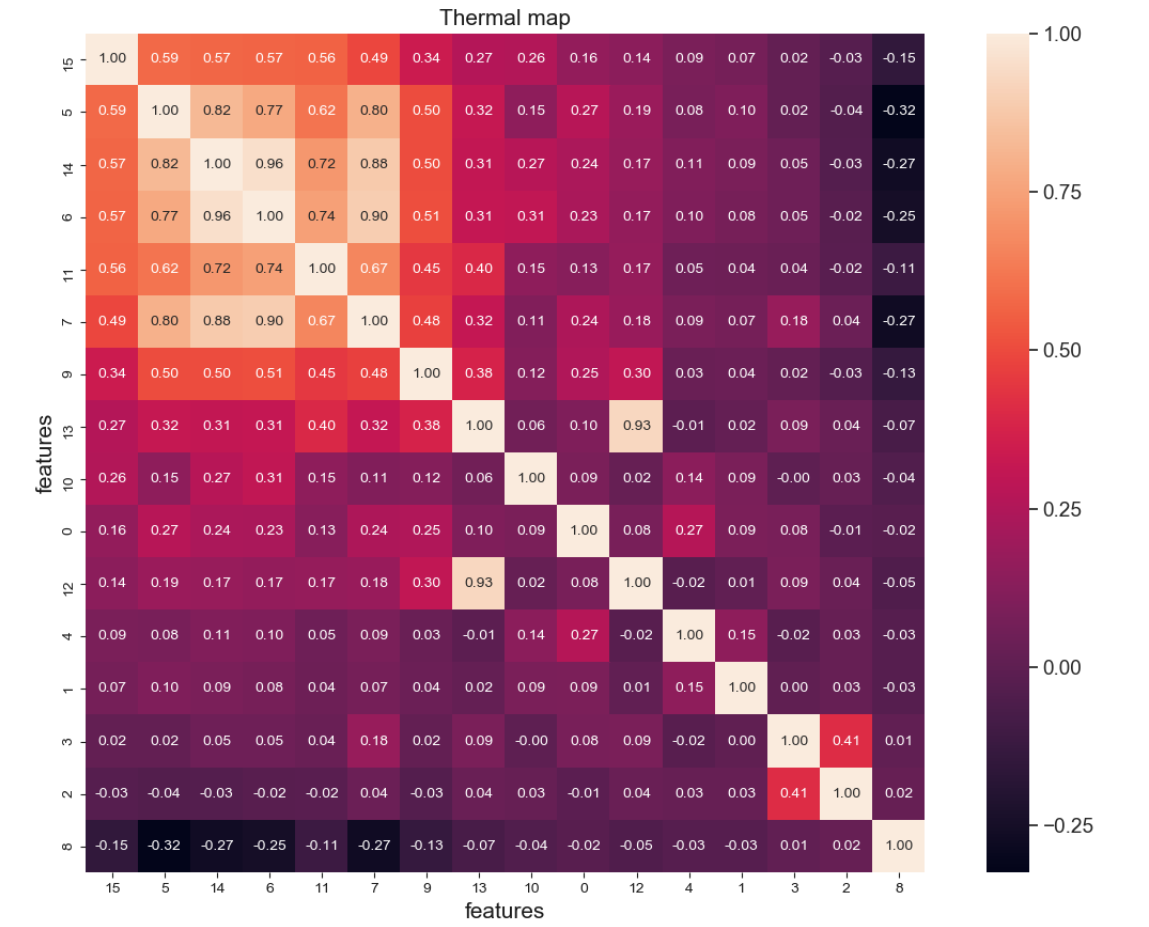3.雷达图（聚类特征描述）

3.1 雷达图说明

1.雷达图作用是把信息在多个轴上进行评估，从而知道哪个轴数据表现优秀

2.还可用于对聚类后结果的特征描述。

3.2 函数接口

正常的plot图像不作过多解释，直接上代码

3.3 雷达图方法一

import pandas as pd
import numpy as np
import seaborn as sns
From sklearn.cluster import KMeans

# ==========绘制聚类结果雷达图

# 构建聚类模型
estimater = KMeans(n_clusters=3)
estimater = estimater.fit(X=data)

# 统计样本属性个数
N = len(estimater.cluster_centers_)

# 将360度N等分，注意：要首位相接
angles = np.linspace(0, 2*np.pi, N, endpoint=False)
angles = np.concatenate((angles, [angles]))

# 画布上构建子图
fig = plt.figure(figsize=(7, 7))
Sam = ["r", "g", "b"]

# 待显示的图例标签
lab = []

# 遍历所有聚类中心
for i in range(len(estimater.cluster_centers_)):
values = estimater.cluster_centers_[i]
features = data.columns
values = np.concatenate((values, [values]))
ax.plot(angles, values, Sam[i], linewidth=2)

# 颜色填充
# ax.fill(angles, values, alpha=0.25)

# 摆放特征名称
ax.set_thetagrids(angles*180/np.pi, features)

# 显示轴网络
ax.grid(True)
lab.append("customer"+str(i))
plt.title("title")

# 显示图例
plt.legend(lab)
plt.show()



3.4 雷达图方法二

pygal

一个python svg图形绘图库。免费的第三方库，可自由进行修改，但没有任何保证。内部提供的是一些绘图的接口（条形图，堆积条形图，点状图，漏斗图，箱型图，仪表图，实心仪表，直方图，水平条形图，水平堆积条形图，线性图，水平线图，堆积线图，水平堆积线图，饼图，水平金字塔图，垂直金字塔图，雷达图，日期横坐标xy图，DateTime横坐标xy图，时间横坐标xy图，TimeDelta横坐标xy图，树状图，XY线图，），可直接进行绘图，相对于pyplot要简便一些。

import matplotlib.pyplot as plt
import pandas as pd
import pygal as pg

centers = [[55.46, 0.82, 30.06],
[10.33, 0.88, 30.18],
[10.71, 0.75, 29.84]]
df_features = pd.DataFrame(centers)
df_features.columns = ["a", "b", "c"]
df_features.index = ["aa", "bb", "cc"]

# 构建雷达图对象

# 设置雷达图标题

# 设置雷达图x轴标签

# 在图中添加对象

# 将图写入文件（可浏览器打开）
radar_chart.render_to_file('bar_chart.svg')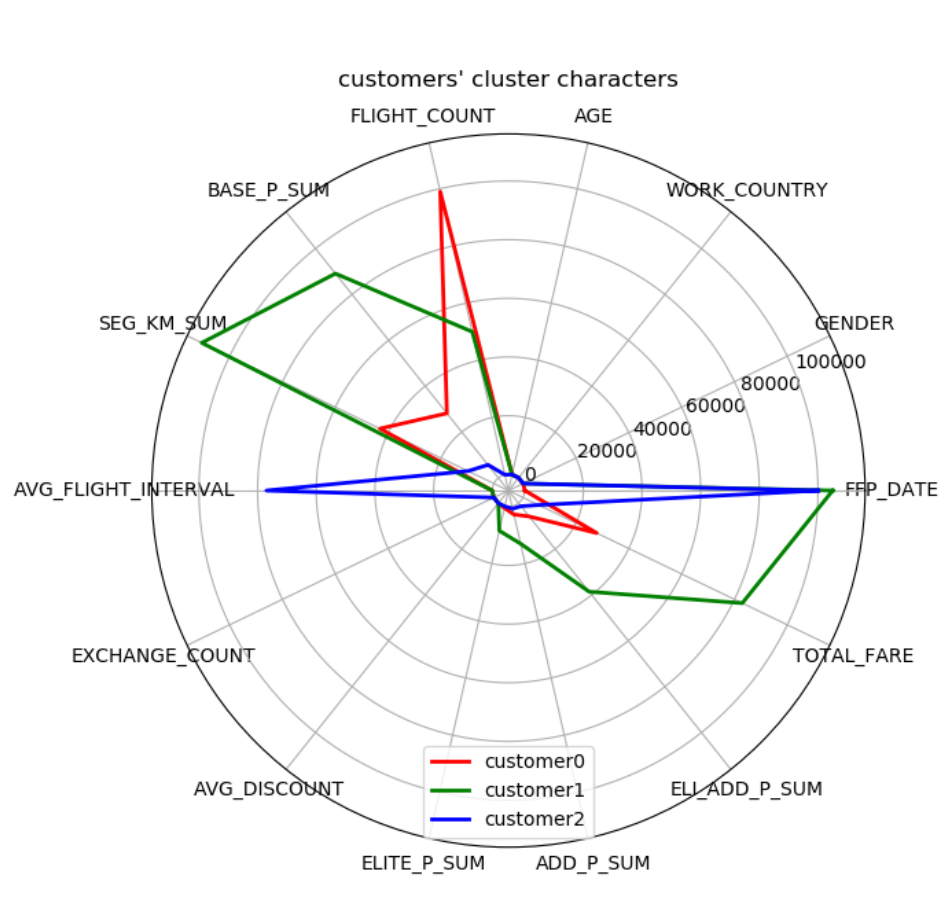4.箱线图

https://www.jianshu.com/p/b2f70f867a4a

4.1 箱线图简介

箱线图是一种用作显示一组数据分散情况的统计图。常用于品质管理。主要用于反应原始数据的分布特征，还可进行多组数据分布特征的比较。从箱线图中可找出最大值、最小值、中位数、下四分位数(25%)、上四分位数(75%)、异常值。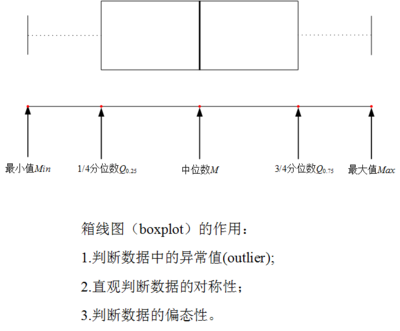注意：区间[Q1-1.5△Q, Q3+1.5△Q]之外的数据值被认为是异常值，应当被忽略。

4.2 第一种实现方式

通过matplotlib.pyplot实现

plt.boxplot(x, whis=None, labels=None)

参数：

x: 输入数据。数组(array)或容器的序列(a sequence of vectors)

whis: 浮点型，序列、字符串（默认为1.5）。调整的是判断异常值式(Q3-Q1)所占的权重

labels: 序列(sequence)。并且，长度必须与数据集的维度相同。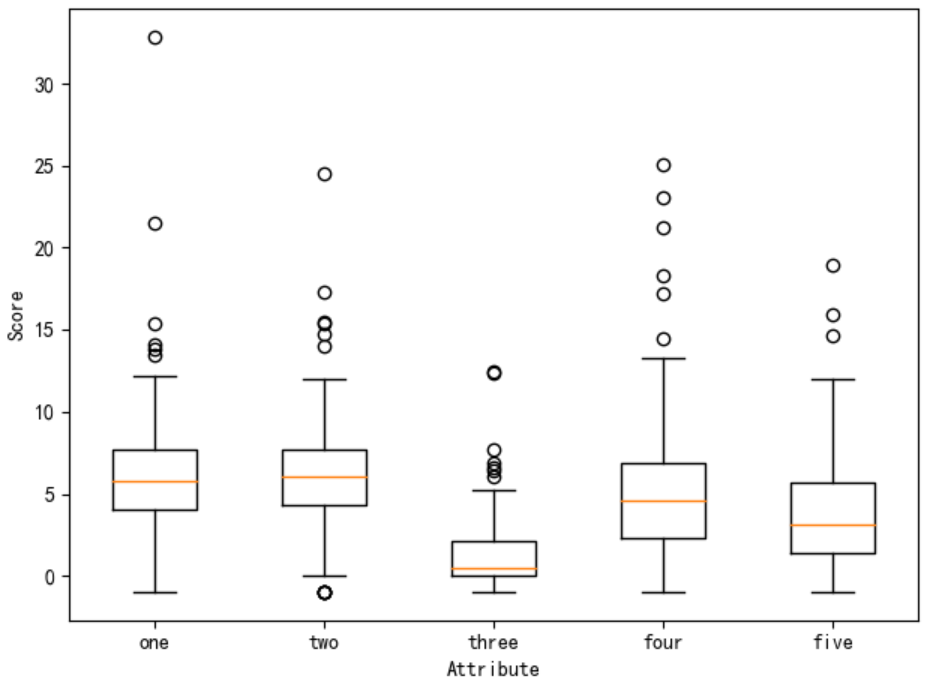import matplotlib.pyplot as plt
import pandas as pd

array = dataFile.iloc[:, 10:15].values

plt.rcParams["axes.unicode_minus"] = False
plt.rcParams["font.sans-serif"] = "SimHei"

plt.boxplot(x=array, labels=["one", "two", "three", "four", "five"], whis=1.5)
plt.xlabel("Attribute")
plt.ylabel("Score")
plt.show()

4.3 第二种实现方式

pandas自带的画图工具(图片感觉不美观)

DataFrame.boxplot(grouped, subplots=True, column=None, fontsize=None, rot=0, grid=True, ax=None, figsize=None, layout=None, **kwds)

plt.show()

参数：

column: 待绘制箱线图的属性列。列名(字符串)、列表、容器。

fontsize: 图中文字大小。整形(int)或字符型(str)

rot: xlabels倾斜的角度。整形(int: 正负均可)、浮点型(float)、字符串(str)

grid: 是否显示图片中的网格。默认为True

figsize: 图片大小。元组 -> (长，宽)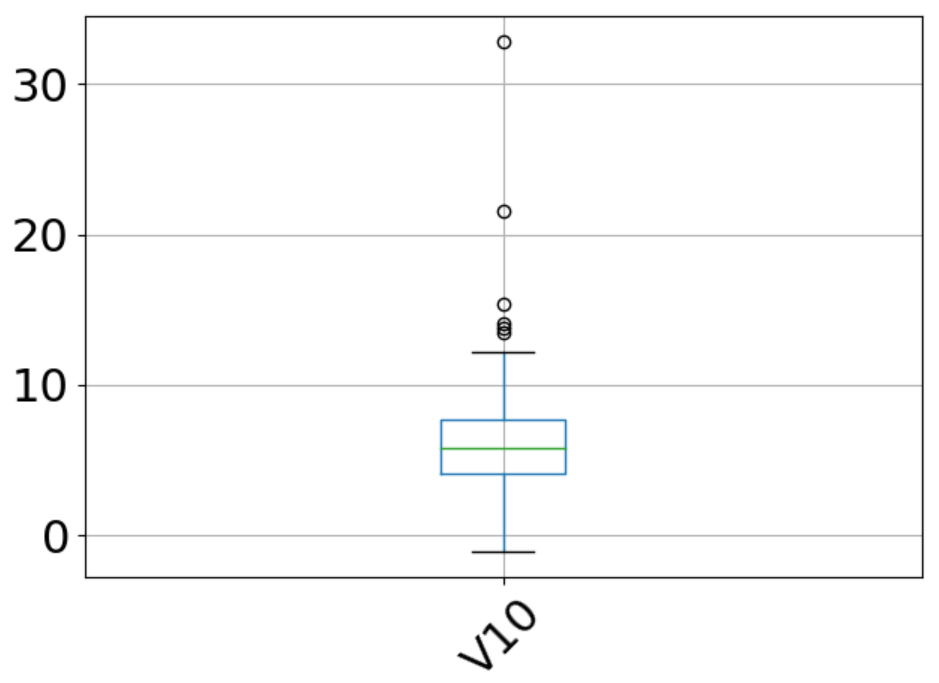import pandas as pd
import matplotlib.pyplot as plt

dataFile = dataFile.iloc[:, 10:15]
dataFile.boxplot(column="V10", fontsize="22", rot=45)
plt.show()

4.4 第三种方式实现

from pylab import *

boxplot(x, whis=None, labels=None)

参数：

x: 绘制箱线图的属性列数据。array

whis: 判断异常值时的权重 [Q1-whis * △Q, Q3+whis * △Q]

labels: xlabels的名称。列表，长度应当与绘图数据的属性个数相同

show()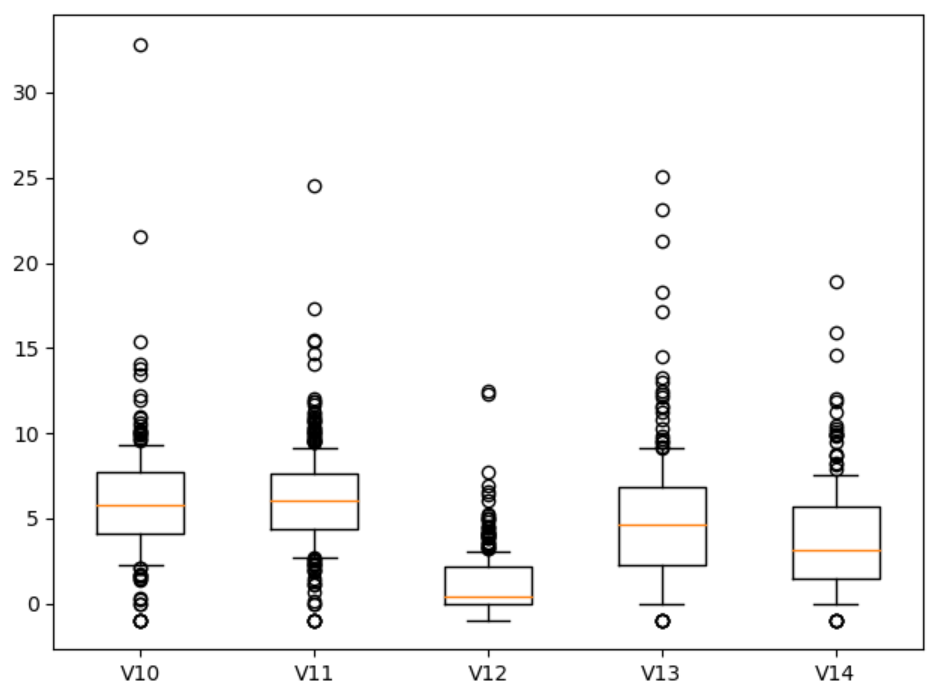from pylab import *
import matplotlib.pyplot as plt
import pandas as pd

dataFile = dataFile.iloc[:, 10:15]

boxplot(dataFile.values, whis=0.5, labels=["V10", "V11", "V12", "V13", "V14"])
show()

5. 平行坐标

平行坐标是一种比较常见的可视化方法，用于对高纬集合和多元数据的可视化。平行坐标为了表示在高纬空间中的一个点集，在N条平行的线的背景下（一般这N条线都竖直且等距），一个在高纬空间的点被表示为一条拐点在N条平行坐标轴的折线，在第K个坐标轴的位置就表示这个点在第K纬的值，平行坐标是信息可视化的一种重要技术。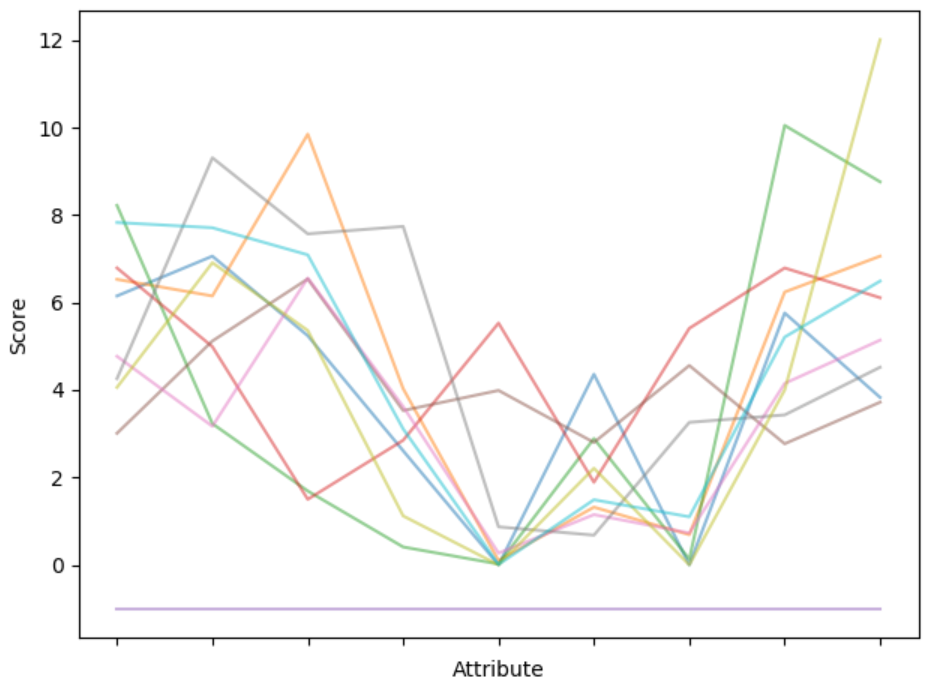import matplotlib.pyplot as plt
import pandas as pd
import numpy as np

summary = df_features.describe()
minRings = -1
maxRings = 99
nrows = 10

for i in range(nrows):
dataRow = df_features.iloc[i, 1:10]
labelColor = (df_features.iloc[i, 10] - minRings)/(maxRings - minRings)
# dataRow.plot(colors=plt.cm.RdY1Bu(labelColor), alpha=0.5)
dataRow.plot(alpha=0.5)

plt.xlabel("Attribute")
plt.ylabel("Score")
plt.show()

6.3D表面图

6.1 所需第三方库

from mpl_toolkits.mplot3d import Axes3D

mplot3d模块中的Axes3D绘图类

from matplotlib.ticker import LinearLocator, FormatStrFormatter

ticker模块主要提供“刻度的划分”和“刻度值的格式化”

matplotlib包中ticker模块中LinearLocator类（刻度划分）和FormatStrFormatter类（使用“%”来格式化轴刻度值）from matplotlib import cm

matplot内置的色彩映射模块

6.2 重点函数

①ax = Axes3D(fig)：将matplotlib.figure.Figure对象转换成rect=[left, bottom, width, height]的3D对象

②np.meshgrid(*xi, indexing, sparse, copy, **kwargs)：将坐标向量转换成坐标矩阵

【参数】

*xi：表示网格坐标的一维数组

indexing：设置输出索引的形式。（笛卡尔("xy")和矩阵("ij")）

sparse: 默认为False.若为True,则为节省内存返回稀疏网格

copy: 默认为True.若为False, 则为节省内存返回原始数组。(sparse=False, copy=False很有可能返回非连续数组)

【调整结果展示】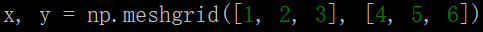①x1：向下拓展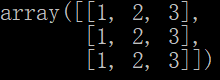②x2:向右拓展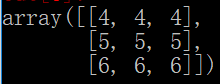③组合成(x1, x2)坐标，并将其定位；

④将(x1, x2)带入f中求解出对应的Z轴数值

③Axes3d.plot_surface(x, y, z, rcount, ccount, rstride, cstride, color, cmap, facecolors, norm, vmin, vmax, shade, *kwargs): 创建表面图

【参数】

x, y, z: 二维数组

rcount, ccount: 每个方向上的最大样本数。默认为50

rstride, cstride: 同rcount 和 ccount的作用都是为了精简坐标轴不同方向的样本数量，因此不能同时设置。默认为10.

color: 表面贴片的颜色surface patches

cmap: 表面贴片的颜色映射 Colormap

facecolors: 每个小块的颜色（list形式）

norm, vmin, vmax: 颜色映射标准化,标准化的下限和上限

linewidth: 线条的宽度

antialiased： 是否平滑(True or False)

# ==========python绘制3D表面图（3维图）
# class Axes3D: 3D绘图类
# module ticker： 提供 ”刻度的定位“ 及 ”刻度的格式化“
# class LinearLocator: 刻度划分
# class FormatStrFormatter: 使用“%”来格式化轴刻度值
# module cm: 内置的色彩映射模块
from mpl_toolkits.mplot3d import Axes3D
import matplotlib.pyplot as plt
from matplotlib.ticker import LinearLocator, FormatStrFormatter
import numpy as np
from matplotlib import cm

fig = plt.figure(figsize=(10, 8))

# 将matplotlib.figure.Figure对象转换成rect=[left, bottom, width, height]的3D对象
ax = Axes3D(fig=fig)

X = np.arange(-5, 5, step=0.1)
Y = np.arange(-5, 5, step=0.1)

# np.meshgrid: 坐标向量转变为坐标矩阵
X, Y = np.meshgrid(X, Y, indexing="xy", sparse=True, )
R = np.sqrt(X**2 + Y**2)
Z = np.cos(R)

# ax.plot_surface: 创建表面图
# ax.set_zlim: 设置3D图中z轴的数值
# ax.zaxis: 初始化3D轴
# LinearLocator(numticks=10): 将z轴刻度划分为10部分
# FormatStrFormatter("%.2f")：z轴刻度值表里路两位小数
surf = ax.plot_surface(X, Y, Z, rstride=1, cstride=1, cmap=plt.get_cmap("rainbow"), linewidth=0, antialiased=False)
ax.set_zlim(-1.01, 1.01)
ax.xaxis.set_major_locator(LinearLocator(numticks=10))
ax.xaxis.set_major_formatter(FormatStrFormatter("%.1f"))
ax.yaxis.set_major_locator(LinearLocator(numticks=10))
ax.yaxis.set_major_formatter(FormatStrFormatter("%.1f"))
ax.zaxis.set_major_locator(LinearLocator(numticks=10))
ax.zaxis.set_major_formatter(FormatStrFormatter("%.1f"))

# fig.colorbar: 创建颜色栏
# shrink: 颜色条长度的收缩比
# aspect: 颜色条宽度
fig.colorbar(surf, aspect=5, shrink=0.5)
plt.show()

7.绘制混淆矩阵

混淆矩阵是机器学习当中一中评价模型分类效果的方法，通过决策矩阵就可计算出单分类的准确率（accuracy）、查全率、查准率、召回率（recall_score）和F1-score等。

from sklearn.metrics import confusion_matrix    # 生成混淆矩阵函数
import matplotlib.pyplot as plt    # 绘图库
import numpy as np
import tensorflow as tf

def plot_confusion_matrix(cm, labels_name, title):
# 归一化
cm = cm.astype('float') / cm.sum(axis=1)[:, np.newaxis]
# 在特定的窗口上显示图像
plt.imshow(cm, interpolation='nearest')
plt.title(title)

# 标度条
plt.colorbar()

num_local = np.array(range(len(labels_name)))
# 设定x轴标签
# rotation:为标签的旋转角度
plt.xticks(num_local, labels_name, rotation=90)
plt.yticks(num_local, labels_name)
plt.ylabel('True label')
plt.xlabel('Predicted label')

plt.show()

# 此处混淆矩阵为模型实际生成值，因此，此处只给出模型的计算结果
pred_y = session.run(tf.argmax(y_, 1), feed_dict={X: test_x})
cm = confusion_matrix(np.argmax(test_y, 1), pred_y,)
print(cm)
# [[100   1   0   1   6   0   0]
#  [  2 111   3   0   2   1  24]
#  [  0   2  68   5   4   3   2]
#  [  2   0   1 120   7  26   0]
#  [  2   5   3   2 120  11  14]
#  [  2   0   2  12   8 115   1]
#  [  2  25   0   1  14   4 302]]

# 调用绘制混淆矩阵的函数
plot_confusion_matrix(cm, labels_name, "HAR Confusion Matrix")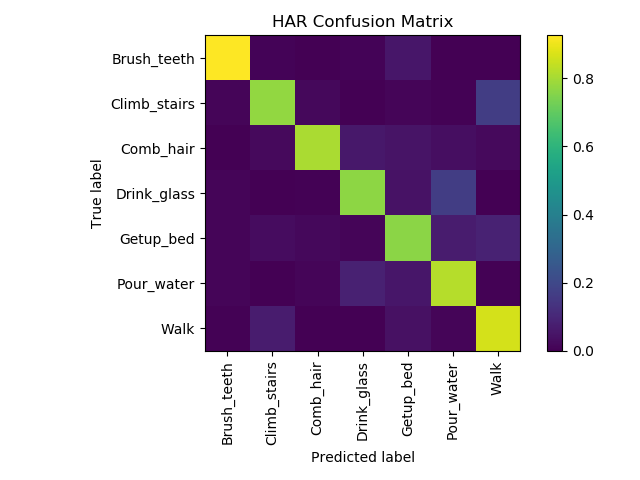8.饼状图

import matplotlib.pyplot as plt
import numpy as np

plt.rcParams["font.sans-serif"] = ["SimHei"]
plt.rcParams["axes.unicode_minus"] = False

labels = ["鸭子", "狗子", "牛子", "猪子"]
values = [15, 30, 45, 10]
explode = (0, 0.1, 0, 0)

plt.figure(figsize=(6, 3))
plt.pie(x=values, explode=explode, labels=labels, autopct="%1.1f")

# 获取或设置轴属性的便捷方法
# equal: 保持图形的形状，不会随着x或者y的拉伸而拉伸
plt.axis("equal")

plt.show()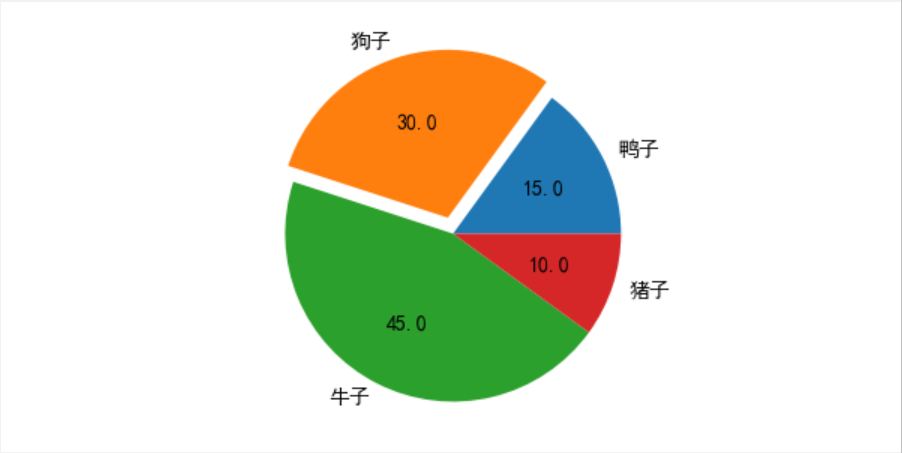9.3D散点图

from mpl_toolkits.mplot3d import Axes3D

mplot3d模块中的Axes3D绘图类

from matplotlib.ticker import LinearLocator, FormatStrFormatter

ticker模块主要提供“刻度的划分”和“刻度值的格式化”

matplotlib包中ticker模块中LinearLocator类（刻度划分）和FormatStrFormatter类（使用“%”来格式化轴刻度值）from matplotlib import cm

matplot内置的色彩映射模块

# ! /usr/bin/env python
# coding:utf-8
# python interpreter:3.6.2
import matplotlib.pyplot as plt
import pandas as pd
import numpy as np
from sklearn.cluster import KMeans
from sklearn import preprocessing
from mpl_toolkits.mplot3d import Axes3D
from matplotlib import cm
plt.rcParams["font.sans-serif"] = ["SimHei"]  # 设置显示字体
plt.rcParams["axes.unicode_minus"] = False  # 设置正常显示符号

X = data
# X = preprocessing.minmax_scale(X)
# X = pd.DataFrame(X)

# 构造聚类模型
estimator = KMeans(n_clusters=3)
estimator.fit(X)
label_pred = estimator.labels_

# 绘制k-means结果
x0 = X[label_pred == 0]
x1 = X[label_pred == 1]
x2 = X[label_pred == 2]
# x3 = X[label_pred == 3]

# 绘制三维散点图
fig = plt.figure()
ax = Axes3D(fig)

# x，y,s要求是list等的array_list类型的数据
ax.scatter(list(x0.iloc[:, 0]), list(x0.iloc[:, 1]), list(x0.iloc[:, 2]), c="red", marker='o', label='label0')
ax.scatter(list(x1.iloc[:, 0]), list(x1.iloc[:, 1]), list(x1.iloc[:, 2]), c="green", marker='*', label='label1')
ax.scatter(list(x2.iloc[:, 0]), list(x2.iloc[:, 1]), list(x2.iloc[:, 2]), c="blue", marker='+', label='label2')
# ax.scatter(list(x3.iloc[:, 0]), list(x3.iloc[:, 1]), list(x3.iloc[:, 2]), c="pink", marker='x', label='label3')

ax.set_xlabel('属性个数')
ax.set_ylabel('标签个数')
ax.set_zlabel('样本量')

data["label"] = "0"
for i in range(data.shape):
data.iloc[i, 3] = list(estimator.labels_)[i]

plt.legend()
plt.show()


展开全文箱线图 平行坐标
• 显然cv::SparseMat存储更为节省空间，典型使用cv::SparseMat的例如直方数据的存储。 1 创建与初始化cv::Mat  cv::Mat用于密集连续的n维数据存储。成员变量： flags： 数据内容标识； dims： 数据维数...
三、大型数组类

OpenCV3对大型数据的存储，具有代表性的是 cv::Mat和cv::SparseMat 类型。 cv::Mat针对的是密集连续性的存储，大多数的图像数据被存储为这种类，即使数据为空，预留的存储空间仍然存在；而cv::SparseMat针对的是稀疏的存储方式，只有数据不为0才保留空间，否则不会预留。显然cv::SparseMat存储更为节省空间，典型使用cv::SparseMat的例如直方图数据的存储。

1 创建与初始化cv::Mat

cv::Mat用于密集连续型的n维数据存储。成员变量：

flags： 数据内容标识；
dims： 数据维数；
rows和cols： 数据行列数；
data： 指向存储的数据；
refcount: 用于智能指针的引用计数。
cv::Mat分为头部和数据部分，不拷贝数据的操作为“浅拷贝”，只是复制了头部；如果拷贝了数据则叫“深拷贝”，这种操作会创建空间并拷贝对方的数据。

m.row(j)返回一个Mat类型，m.row(j).copyTo(m.row(i))，可以用于讲一个Mat 拷贝到另一个Mat 中。

我们可以通过载入图像来创建Mat类型矩阵，当然也可以直接手动创建矩阵，基本方法是指定矩阵尺寸和数据类型：

// 先声明，再创建数据
cv::Mat m;
// Create data area for 3 rows and 10 columns of 3-channel 32-bit floats
m.create( 30, 20, CV_32FC3 );
// Set the values in the 1st channel to 1.0, the 2nd to 0.0, and the 3rd to 1.0
m.setTo( cv::Scalar( 255.0f, 0.0f, 255.0f ) );

// 同时创建
cv::Mat m( 3, 10, CV_32FC3, cv::Scalar( 255.0f, 0.0f, 255.0f ) );

//
cv::Mat a(cv::Size(5,5),CV_8UC1); // 单通道
cv::Mat b = cv::Mat(cv::Size(5,5),CV_8UC3); //3通道每个矩阵元素包含3个uchar值

// 初始化方法
cv::Mat mz = cv::Mat::zeros(cv::Size(5,5),CV_8UC1); // 全零矩阵
cv::Mat mo = cv::Mat::ones(cv::Size(5,5),CV_8UC1);  // 全1矩阵
cv::Mat me = cv::Mat::eye(cv::Size(5,5),CV_32FC1);  // 对角线为1的对角矩阵



结果：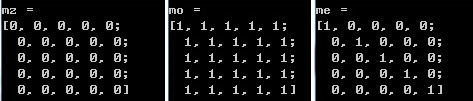其中，type的组成方式为：CV_{8U,16S,16U,32S,32F,64F}C{1,2,3} 。例如：CV_32FC3 代表32bit浮点类型的三通道数据。图像处理中常用的几种数据类型如下：

CV_8UC1// 8位无符号单通道
CV_8UC3// 8位无符号3通道
CV_8UC4
CV_32FC1// 32位浮点型单通道
CV_32FC3// 32位浮点型3通道
CV_32FC4


包括数据位深度8位、32位，数据类型U:uchar、F:float型以及通道数C1：单通道、C3：三通道、C4：四通道。

type函数

opencv中Mat存在各种类型，其中mat有一个type（）的函数可以返回该Mat的类型。类型表示了矩阵中元素的类型以及矩阵的通道个数，它是一系列的预定义的常量，其命名规则为CV_(位数）+（数据类型）+（通道数）。具体的有以下值：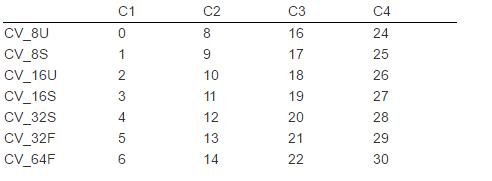2 元素访问

cv::Mat元素的方式：位置访问、迭代器访问和块访问。

2.1 位置访问

最直接的位置访问方式是使用 at<>() ：

cv::Mat m = cv::Mat::eye( 10, 10, 32FC1 );//创建单位阵
printf("Element (3,3) is %f\n",m.at<float>(3,3));

cv::Mat m = cv::Mat::eye( 10, 10, 32FC2 );
printf("Element (3,3) is (%f,%f)\n",m.at<cv::Vec2f>(3,3),m.at<cv::Vec2f>(3,3));

2.2 迭代器访问

使用 cv::MatIterator<> 和cv::MatConstIterator<> 迭代器可以对cv::Mat元素进行访问：

int sz = { 4, 4, 4 };
cv::Mat m( 3, sz, CV_32FC3 ); // A three-dimensional array of size 4-by-4-by-4
cv::randu( m, -1.0f, 1.0f ); // fill with random numbers from -1.0 to 1.0
float max = 0.0f; // minimum possible value of L2 norm

cv::MatConstIterator<cv::Vec3f> it = m.begin();
while( it != m.end() )
{
len2 = (*it)*(*it)+(*it)*(*it)+(*it)*(*it);
if( len2 > max ) max = len2;
it++;
}


2.3 块访问

块访问提供对cv::Mat子数组的访问方式。这种访问方式返回的往往是个数组或范围，而不是单个元素。

m.row( i );    //Array corresponding to row i of m
m.col( j );    //Array corresponding to column j of m

m.rowRange( i0, i1 );                         //Array corresponding to rows i0 through i1-1 of matrix m
m.rowRange( cv::Range( i0, i1 ) );    //Array corresponding to rows i0 through i1-1 of matrix m
m.colRange( j0, j1 );                         //Array corresponding to columns j0 through j1-1 of matrix m
m.colRange( cv::Range( j0, j1 ) );    //Array corresponding to columns j0 through j1-1 of matrix m

函数原型：Mat cv::Mat::colRange(int startcol, int endcol) const

功能：该方法为矩阵的指定列跨度创建一个矩阵头。 类似于Mat :: row和Mat :: col，这是一个O（1）操作

参数：（分别表示我们想取的Mat的第几列到第几列）

startcol:一个包含0的起始索引的列跨度。
endcol:一个除0以外的结束索引的列跨度。
m.diag( d );//Array corresponding to the d-offset diagonal of matrix m

m( cv::Rect(i0,i1,w,h) );    //Array corresponding to the subrectangle of matrix m with one cornerat i0, j0 and the opposite corner at (i0+w-1, j0+h-1)

3、矩阵运算

矩阵加减法

我们可以使用"+"和"-"符号进行矩阵加减运算。

cv::Mat a= Mat::eye(Size(3,2), CV_32F);
cv::Mat b= Mat::ones(Size(3,2), CV_32F);
cv::Mat c= a+b;
cv::Mat d= a-b;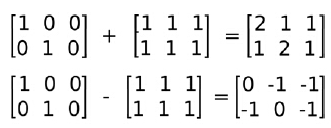矩阵乘法

使用"*"号计算矩阵与标量相乘，矩阵与矩阵相乘（必须满足矩阵相乘的行列数对应规则）

	Mat m1= Mat::eye(2,3, CV_32F); //使用cv命名空间可省略cv::前缀，下同
Mat m2= Mat::ones(3,2, CV_32F);
cout<<"m1  = "<<endl<<m1<<endl<<endl;
cout<<"m2  = "<<endl<<m2<<endl<<endl;
// Scalar by matrix
cout << "\nm1.*2 = \n" << m1*2 << endl;
// matrix per element multiplication
cout << "\n(m1+2).*(m1+3) = \n" << (m1+1).mul(m1+3) << endl;
// Matrix multiplication
cout << "\nm1*m2 = \n" << m1*m2 << endl;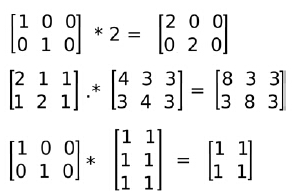矩阵转置

矩阵转置是将矩阵的行与列顺序对调（第i行转变为第i列）形成一个新的矩阵。OpenCV通过Mat类的t()函数实现。

// 转置
Mat m1= Mat::eye(2,3, CV_32F);
Mat m1t = m1.t();
cout<<"m1  = "<<endl<<m1<<endl<<endl;
cout<<"m1t  = "<<endl<<m1t<<endl<<endl;
system("pause");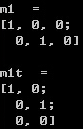求逆矩阵

逆矩阵在某些算法中经常出现，在OpenCV中通过Mat类的inv()方法实现

// 求逆
Mat meinv = me.inv();
cout<<"me  = "<<endl<<me<<endl<<endl;
cout<<"meinv = "<<endl<<meinv<<endl<<endl;
system("pause");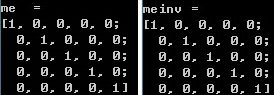计算矩阵非零元素个数

计算物体的像素或面积常需要用到计算矩阵中的非零元素个数，OpenCV中使用countNonZero()函数实现。

// 非零元素个数
int nonZerosNum = countNonZero(me); // me为输入矩阵或图像
cout<<"me  = "<<endl<<me<<endl;
cout<<"me中非零元素个数 = "<<nonZerosNum<<endl<<endl;
system("pause");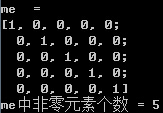均值和标准差

OpenCV提供了矩阵均值和标准差计算功能，可以使用meanStdDev(src,mean,stddev)函数实现。

参数

src – 输入矩阵或图像
mean – 均值，OutputArray
stddev – 标准差，OutputArray

// 均值方差
Mat mean;
Mat stddev;
meanStdDev(me, mean, stddev); //me为前文定义的5×5对角阵
cout<<"mean = "<<mean<<endl;
cout<<"stddev = "<<stddev<<endl;
system("pause");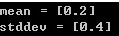需要说明的是，如果src是多通道图像或多维矩阵，则函数分别计算不同通道的均值与标准差，因此返回值mean和stddev为对应维度的向量

	Mat mean3;
Mat stddev3;
Mat m3(cv::Size(5,5),CV_8UC3,Scalar(255,200,100));
cout<<"m3  = "<<endl<<m3<<endl<<endl;
meanStdDev(m3, mean3, stddev3);
cout<<"mean3 = "<<mean3<<endl;
cout<<"stddev3 = "<<stddev3<<endl;
system("pause");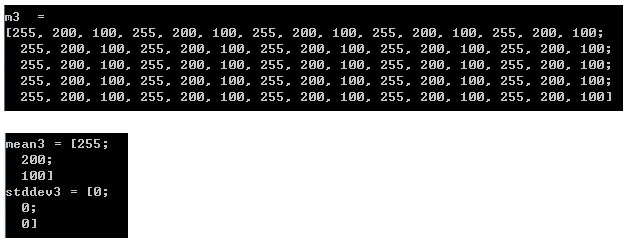求最大最小值

求输入矩阵的全局最大最小值及其位置，可使用函数minMaxLoc();

void minMaxLoc(InputArray src, CV_OUT double* minVal,
CV_OUT double* maxVal=0, CV_OUT Point* minLoc=0,


参数：

src – 输入单通道矩阵（图像）.
minVal – 指向最小值的指针， 如果未指定则使用NULL
maxVal – 指向最大值的指针， 如果未指定则使用NULL
minLoc – 指向最小值位置（2维情况）的指针， 如果未指定则使用NULL
maxLoc – 指向最大值位置（2维情况）的指针， 如果未指定则使用NULL
from:https://blog.csdn.net/iracer/article/details/51296631

cv::Mat支持的其他操作：

m1 = m0.clone();	//（深拷贝）Make a complete copy of m0, copying all data elements as well; cloned array willbe continuous
m0.copyTo( m1 );	//equivalent to m1=m0.clone()
m0.copyTo( m1, mask );	//Same as m0.copyTo(m1), except only entries indicated in the array mask arecopied
m0.convertTo(m1, type, scale, offset);	//在缩放或不缩放的情况下转换为另一种数据类型。

m0.setTo( s, mask );	//将阵列中所有的或部分的元素设置为指定的值; if mask is present, set only those valuescorresponding to nonzero elements in mask
m0.reshape( chan, rows );	//在无需复制数据的前提下改变2D矩阵的形状和通道数或其中之一。

m0.push_back( s );	//Extend an m × 1 matrix and insert the singleton s at the end
m0.push_back( m1 );	//Extend an m × n by k rows and copy m1 into those rows; m1 must be k × n
m0.pop_back( n );	Remove n rows from the end of an m × n (default value of n is 1)

m0.locateROI( size, offset );	Write whole size of m0 to cv::Size size; if m0 is a “view” of a larger matrix,write location of starting corner to Point& offset
m0.adjustROI( t, b, l, r );	Increase the size of a view by t pixels above, b pixels below, l pixels to the left,and r pixels to the right
m0.total();	Compute the total number of array elements (does not include channels)
m0.isContinuous();	Return true only if the rows in m0 are packed without space between them inmemory

m0.elemSize();	//Return the size of the elements of m0 in bytes (e.g., a three-channel float matrixwould return 12 bytes)
m0.elemSize1();	//Return the size of the subelements of m0 in bytes (e.g., a three-channel floatmatrix would return 4 bytes)

m0.type();	//Return a valid type identifier for the elements of m0 (e.g., CV_32FC3)
m0.depth();	//Return a valid type identifier for the individial channels of m0 (e.g., CV_32F)
m0.channels();	//Return the number of channels in the elements of m0
m0.size();	//Return the size of the m0 as a cv::Size object
m0.empty();	//Return true only if the array has no elements (i.e., m0.total==0 orm0.data==NULL)


推荐一个写的很好的Mat矩阵的博客：https://blog.csdn.net/giantchen547792075/article/details/7169255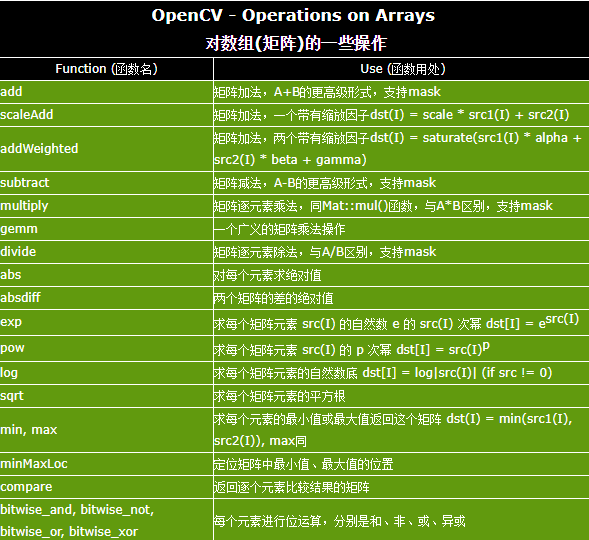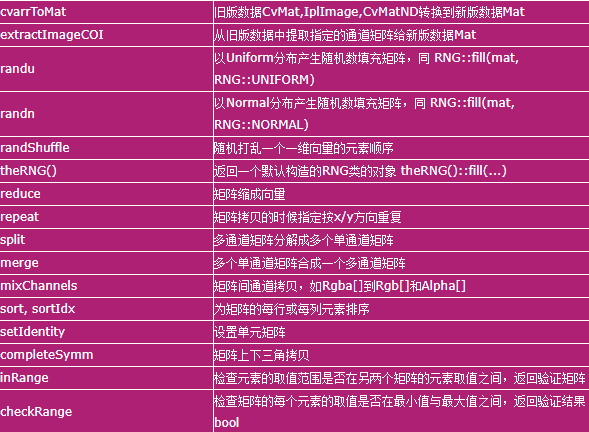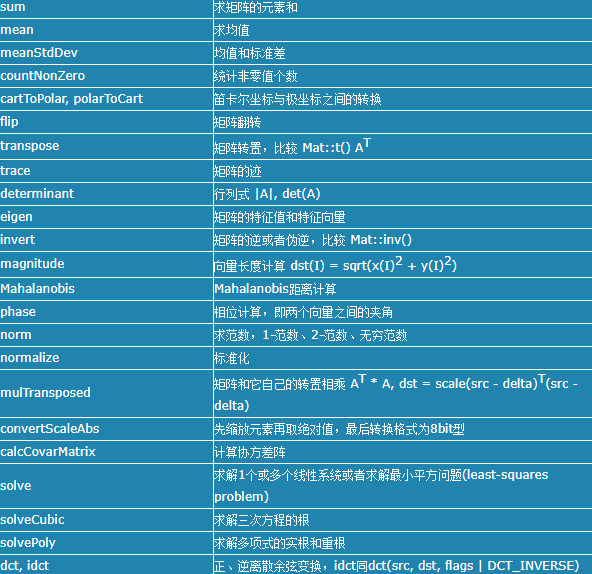from:http://blog.sina.com.cn/s/blog_7908e1290101i97z.htm

展开全文opencv
• 1、Mat矩阵点乘——A*B Opencv重载了运算符“*”，姑且称之为Mat矩阵“点乘”，其中一个重载声明为： [cpp] view plain copy  print? CV_EXPORTS MatExpr operator * (const ...opencv 算法 应用
• 网络中常用的几种矩阵： 1.度矩阵：2.相邻矩阵： 3.拉普拉斯矩阵： 给定一个n个顶点的G，它的拉普拉斯矩阵   定义为: L=D-A 其中D为的度矩阵，A为的邻接矩阵。度矩阵中，只需要考虑出度或者...
• 的代数表示方法通常两种 邻接矩阵 关联矩阵 对于G=(V,E), 点数为n，边数为m； 1. 邻接矩阵A ...行为顶点，列也为顶点 的n*n矩阵矩阵元素aij=vi与vj之间关联的边数...若G为简单，则A(G)是布尔型矩阵；行...
• ## 矩阵

千次阅读 2012-09-09 11:15:21
矩阵 维基百科，自由的百科全书 线性代数 向量 · 矩阵 · 行列式 · 线性空间 显示▼向量 显示▼矩阵与行列式 显示...算法 图像处理 汇编
• ## 矩阵

千次阅读 2009-06-02 09:30:00
英文名Matrix（矩阵）本意是子宫、母体、孕育生命的地方，同时，在数学名词中，矩阵用来表示统计数据等方面的各种关联的数据。这个定义很好地解释了Matrix代码制造世界的数学逻辑基础。　数学上，矩阵就是由方程组...matrix c 算法
•  常用的图形思维导图、跨职能流程、流程和鱼骨头、N-S 等等。下面分别对各个图示及使用方法简单介绍。 思维导图又称脑图，有利于人脑的扩散思维的展开。通常用在整理读书笔记、测试思路、头脑风暴时使用。...测试 软件开发
• 一、Mat初始化 1.使用Mat构造函数 ...//其实是2*6的矩阵，因为每个元素3个通道。 Mat M1( 2, 2, CV_8UC1，Scalar(0) );//单通道 //方法二： int sz = {2, 2, 2}; Mat L( 3, sz, CV_8UC(1), Scalar::all(...
• 矩阵和图像操作 表3-3列出了一些操作矩阵图像的函数，其中的大部分对于图像处理非常有效。它们实现了图像处理中的基本操作，例如对角化、矩阵变换以及一些更复杂的诸如计算图像的统计操作。 【47】 表图像 函数
• 模型评估通常作为建模的最后一步，用于评估模型效果，判别该模型是否...连续性预测模型常用的是MAPE/RMSE，而分类常用AUC/accuracy/recall/specify`等等。本文将介绍常用的评估指标，并对应指出各指标的不同称呼。ROC MAPE
• 读取图像：用imread函数读取图像文件，文件格式可以是TIFF、JPEG、GIF、BMP、PNG等。...如果图片是彩色的，可以用rgb2gray转换成灰度： 　 　>> f = rgb2gray(f); 　然后可以用size函数看图像的大小
• ## 非负矩阵分解NMF

万次阅读 多人点赞 2016-08-03 12:37:26
http://blog.csdn.net/pipisorry/article/details/52098864非负矩阵分解(NMF,Non-negative matrix factorization)NMF的发展及原理　著名的科学杂志《Nature》于...该文提出了一种新的矩阵分解思想——非负矩阵分解(NonPCA
• ## OpenCV—基本矩阵操作与示例

万次阅读 多人点赞 2016-05-02 16:30:14
图像处理中对矩阵的操作非常重要，本文总结了使用OpenCV进行矩阵的创建、初始化以及基本矩阵操作，给出了示例代码。OpenCV C++ 图像处理
• ## 理解矩阵，矩阵背后的现实意义

千次阅读 多人点赞 2018-01-15 16:28:19
这是很早以前已经看过的，最近无意中又把保存的文章翻出来时，想起很多朋友问过矩阵，虽对矩阵似懂非懂，但却很想弄懂它，希望这几篇文章能帮你一下，故转之： 线性代数课程，无论你从行列式入手还是直接从矩阵入手...matrix transform 变换
• 的定义 的存储结构 邻接矩阵 邻接表 对比邻接矩阵与邻接表 十字链表 邻接多重表   前言 在线性表中，数据元素之间仅线性关系，每个数据元素只有一个直接前驱和一个直接后继； 在树形结构中，数据...数据结构
• OpenCV 如何对图像的像素进行操作 ...如果图像是一幅灰度图像，他就像这样，从左到右，从上到下，依次是矩阵的每一行每一列，这时候矩阵M(i,j)的值自然就是当前点的灰度值了。 而对于一幅彩色图像，由于它opencv 内置函数
• 前面，小编和大家一起学习了SPSS Modeler中“图形板”节点，今天咱们一起看看图形板中常用的图形，包括气泡、散点图矩阵、箱式、聚类箱、热图。 1. 气泡 目的：分析连续变量的关联性，可看作是散点的一...
• 在实践过程中，通过测试代码，我发现基于BufferedImage可以两种获取像素矩阵的方式，但需要注意像素点的保存顺序问题。 具体表现在：本例中的图片是一副彩色图片（博客头像），大小为425*292，即宽度（Width）为...图像读取 java
• ## 决策矩阵

千次阅读 2010-06-06 14:10:00
什么是决策矩阵 　决策矩阵是风险决策 常用的分析手段之一，又称“决策表”、“益损矩阵”、“益损表”、“风险矩阵” 。  决策矩阵的应用 　决策矩阵由备选方案、自然状态(及其发生的概率)...工具 框架 c
• 常用的是im2double函数，将 uint8图像转为double类型，范围为0-1，如果是255的图像，那么255转为1，0还是0，中间的做相应改变。 MATLAB中读入图像的数据类型是uint8，而在矩阵中使用的数据类型是double。因此 I2=im2...matlab double
• ## 理解矩阵

万次阅读 多人点赞 2012-09-21 16:08:58
无意中看到孟岩老师的关于理解矩阵的博客，为之思维所惊叹！受益匪浅啊，故转载之：   理解矩阵一： 转载自：http://blog.csdn.net/myan/article/details/647511 前不久chensh出于不可告人的目的，要充当老师，...工具 算法
• ## Matlab常用图像操作

千次阅读 2009-05-02 22:02:00matlab fft 图像处理 存储
• 向量可以被认为是具有n个相互独立的性质（维度）的对象的表示，矩阵又是什么呢？我们如果认为矩阵是一组列（行）向量组成的新的复合向量的展开式，那么为什么这种展开式具有如此广泛的应用？特别是，为什么偏偏二教学
• ## 决策矩阵分析法

千次阅读 2018-11-06 10:26:28
网格分析，也被称为是决策矩阵分析，是由英国管理学家斯图尔特•普提出的一种多因素辅助决策工具。因此该方法也被称为普氏分析或者多因素辅助分析。它是一款非常有效的辅助决策工具，当你面临很多好的项目选择，同时......